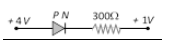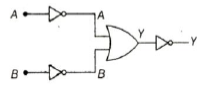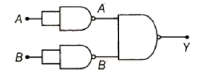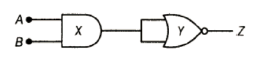## Filters

Sort by :
Clear All
Q
Engineering
139 Views   |

The cut-in voltage for silicon diode is approximately

• Option 1)

0.2V

• Option 2)

0.6V

• Option 3)

1.1V

• Option 4)

1.4V

Knee voltage of P-N junction - It is defined as that forward voltage at which the current through the junction starts rising rapidly with increase in voltage . - wherein Knee voltage for Ge is 0.3 V Knee voltage for Si is 0.7 V   Cut in voltage for silicon diode is approximately 0.6V Option 1) 0.2V This solution is incorrect. Option 2) 0.6V This solution is correct. Option 3) 1.1V This...
Engineering
112 Views   |

In the forward bias arrangement of a PN-junction diode

• Option 1)

The N-end is connected to the positive terminal of the battery

• Option 2)

The P-end is connected to the positive terminal of the battery

• Option 3)

The direction of current is from N-end to P-end in the diode

• Option 4)

The P-end is connected to the negative terminal of battery

As we discussed in concept P -N junction as diode - It is a one way device. It offers a low resistance when forward biased and high resistance when reverse biased. - wherein R = 0, Forward  R  Reverse    In forward biased PN junction diode P type is connected to positive terminal of battery and N type with negative terminal of the battery. Option 1) The N-end is connected to the positive...
Engineering
101 Views   |

In the depletion region of an unbiased P-N junction diode there are

• Option 1)

Only electrons

• Option 2)

Only holes

• Option 3)

Both electrons and holes

• Option 4)

Only fixed ions

As we discussed in concept P-N junction - When p type semiconductor is mixed with N - type semiconductor, P-N junction formed   - wherein There is very thin region (of the order of micro meter) called depution region.    In the depletion region, all the holes and electrons combined together and anhiliate each other and hence only ions will be present in depletion region. Option 1) Only...
Engineering
186 Views   |

In the circuit given below, the value of the current is• Option 1)

• Option 2)

• Option 3)

• Option 4)

As we discussed in concept P -N junction as diode - It is a one way device. It offers a low resistance when forward biased and high resistance when reverse biased. - wherein R = 0, Forward  R  Reverse    From Figure, potential difference = 3V. Since the diode is forward biased resistance offered by diode = 0 Option 1) This option is incorrect. Option 2) This option is correct. Option...
Engineering
99 Views   |

In the circuit below, identify the equivalent gate• Option 1)

OR

• Option 2)

AND

• Option 3)

NOR

• Option 4)

NAND

As we learnt in  AND Gate - - wherein A and B are input Y is out put   Y=A . B This represent AND gate.   Option 1) OR This option is incorrect. Option 2) AND This option is correct. Option 3) NOR This option is incorrect. Option 4) NAND This option is incorrect.
Engineering
92 Views   |

In the circuit below, identify the equivalent gate• Option 1)

OR

• Option 2)

AND

• Option 3)

NOR

• Option 4)

NAND

OR gate - Relation between input and output      - wherein A and B are input Y is out put     D'morgan's Theorem - 1)     2)      3)     4)     - wherein A and B are input.     (D'morgabs law) This represent or gate   Option 1) OR Option is correct Option 2) AND Option is incorrect Option 3) NOR Option is incorrect Option 4) NAND Option is incorrect
Engineering
157 Views   |

In the circuit below, identify the equivalent gate• Option 1)

OR

• Option 2)

XOR

• Option 3)

NOT

• Option 4)

NAND

As we learnt in  NAND gate - NOT + AND gate - wherein A and B are input Y is out put     x= A.B Hence Final output   , represent NAND gate Option 1) OR Option is incorrect Option 2) XOR Option is incorrect Option 3) NOT Option is incorrect Option 4) NAND Option is correct
Engineering
134 Views   |

In forward bias, the width of potential barrier in a P-N junction diode

• Option 1)

Increases

• Option 2)

Decreases

• Option 3)

Remains constant

• Option 4)

First increases then decreases

P -N junction as diode - It is a one way device. It offers a low resistance when forward biased and high resistance when reverse biased. - wherein R = 0, Forward  R  Reverse   In forward bias, The width of potential barrier decreases and hence flow of current becomes high Option 1) Increases  Option is incorrect Option 2) Decreases Option is correct Option 3) Remains constant Option is...
Engineering
101 Views   |

In a PN-junction diode

• Option 1)

The current in the reverse biased condition is generally very small

• Option 2)

The current in the reverse biased condition is small but the forward biased current is independent of the bias voltage

• Option 3)

The reverse biased current is strongly dependent on the applied bias voltage

• Option 4)

The forward biased current is very small in comparison to reverse biased current

P -N junction as diode - It is a one way device. It offers a low resistance when forward biased and high resistance when reverse biased. - wherein R = 0, Forward  R  Reverse   In forward biased condition resistance produced by P-N junction diode is very small and hence current is very large. In Reserve Biased condition ,resistance is very high and hence current is very small Option 1) The...
Engineering
143 Views   |

In a junction diode, the holes are due to

• Option 1)

Protons

• Option 2)

Neutrons

• Option 3)

Extra electrons

• Option 4)

Missing of electrons

p - type semiconductor - Here the base semiconductor (i.e.pure Si or Ge ) is mixed with tetravalent doping material (e.g. Al ) - wherein 1) nh >>ne 2) There is an acceptor level just above  the valence band .     n - type semiconductor - Here the base semiconductor (e.g. pure Si or Ge) is mixed with pentavalent doping material (e.g. Phosphorous) - wherein 1) ne >>nh 2) There is donor energy...
Engineering
259 Views   |

If the forward voltage in a semiconductor diode is doubled, the width of the depletion layer will

• Option 1)

Become half

• Option 2)

Become one-fourth

• Option 3)

Remain unchanged

• Option 4)

Become double

P-N junction - When p type semiconductor is mixed with N - type semiconductor, P-N junction formed   - wherein There is very thin region (of the order of micro meter) called depution region.    width of depletion region  i.e. If V is doubled width of depletion region will become half. Option 1) Become half Correct Option 2) Become one-fourth   Incorrect Option 3) Remain...
Engineering
458 Views   |

A semiconductor device is connected in a series circuit with a battery and a resistance. A current is found to pass through the circuit. If the polarity of the battery is reversed, the current drops almost to zero. The device may be

• Option 1)

A P-type semiconductor

• Option 2)

An N-type semiconductor

• Option 3)

A PN-junction

• Option 4)

An intrinsic semiconductor

P -N junction as diode - It is a one way device. It offers a low resistance when forward biased and high resistance when reverse biased. - wherein R = 0, Forward  R  Reverse    For a PN Junction current easily flow when it is in forward bias and it almost become zero when it is reverse bias. Option 1) A P-type semiconductor Incorrect Option 2) An N-type semiconductor Incorrect Option 3) A...
Engineering
879 Views   |

A PN- junction has a thickness of the order of

• Option 1)

• Option 2)

• Option 3)

• Option 4)

1cm

Exams
Articles
Questions